Organic exercises (2010 VCE) 1) What is the correct systematic name for the following compound?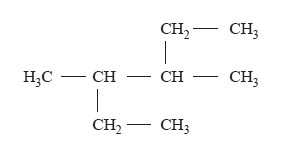A. 2-ethyl-3-methylpentane B. 3-methyl-4-ethylpentane C. 3,4-dimethylhexane D. 2,3-diethylbutane Solution. 2) For which one of the following molecular formulas is there only one possible structure? A. C2HCl3 B. C2H4Cl2 C. C2H2Cl2 D. C4H9OH Solution 3) An organic compound reacts with both dilute hydrochloric acid and dilute sodium hydroxide solution. The compound could be A. C3H7Cl B. C3H7NH2 C. C4H9COOH D. H2NCH2COOH Solution 4) Which one of the following organic reactions does not result in the product shown on the right-hand side of the reaction?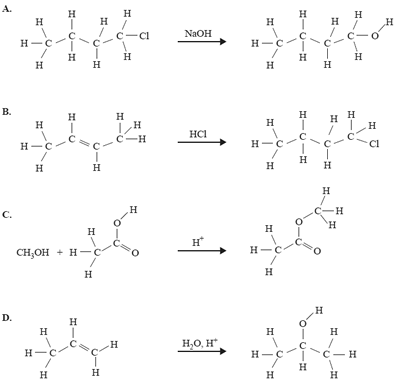Solution5) The following are incomplete and unbalanced equations representing three types of chemical reactions that involve glucose. In reactions 1 and 3, product A is the same compound. In reactions 2 and 3, product B is the same compound. reaction 1 C6H12O6(aq) → C2H5OH(aq) + product A reaction 2 C6H12O6(aq) → C12H22O11(aq) + product B reaction 3 C6H12O6(aq) → product A + product B Which one of the following correctly names reaction 3 and identifies product A and product B?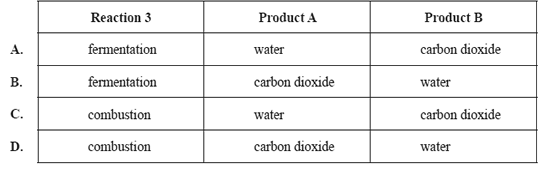Solution6) Copolymers are obtained when two or more different monomers are allowed to polymerise together. Part of a copolymer chain is shown below.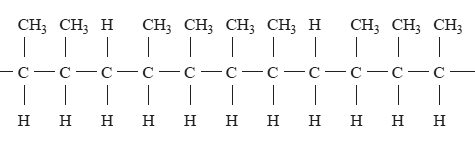The two alkenes that could react together to form this polymer are A. propene and but-1-ene. B. propene and but-2-ene. C. but-1-ene and but-2-ene. D. pent-2-ene and but-2-ene. Solution7) a. Biodiesel is an alternative to standard diesel fuel. Biodiesel is made from biological ingredients instead of petroleum. Biodiesel is usually made from plant oils or animal fats through a series of chemical reactions. In one process a common triglyceride in palm oil, known as POP, is reacted with methanol in the presence of potassium hydroxide as a catalyst. The result is a mixture of methyl esters of the fatty acids (biodiesel). i. The value of the stoichiometric ratio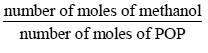is Solution ii. Calculate the volume, in litres, of methanol (density = 0.79 g mL–1) required to react completely with 10.0 kg of the triglyceride POP (Mr = 833) to produce glycerol and the mixture of methyl esters. Solution Click for the assessor's commentsb. Cervonic acid is a polyunsaturated fatty acid found in fish oil. The number of carbon-carbon double bonds in a molecule of cervonic acid can be determined by titration with iodine, I2, solution. An addition reaction takes place between the double bonds in cervonic acid and iodine. 20.00 mL of 0.300 M I2 solution reacted exactly with 0.328 g of cervonic acid. The molar mass of cervonic acid is 328.0 g mol–1. i. Calculate the number of double bonds in a molecule of cervonic acid. Solution Click for the assessor's comments There are 22 carbon atoms in a molecule of cervonic acid. ii. What is the formula of cervonic acid? Solution8) Since ancient times, the bark of willow trees has been used for pain relief. In the 19th century, chemists isolated the active compound, salicin, from the bark. This was eventually converted into aspirin, which is now a widely used drug. The reaction scheme below shows the steps used to carry out the conversion.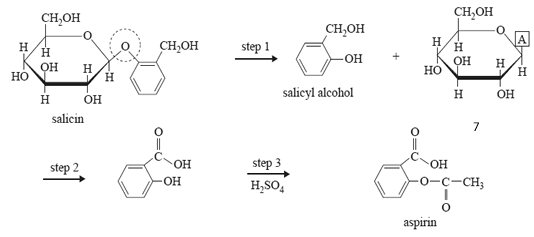a. What type of linkage is circled in the structure of salicin? (1 mark) Solution b. In step 1, salicyl alcohol and another compound is produced. i. What group of biomolecules does this other compound belong to? Solution ii. The structure of this other compound is not complete. Write the formula of the atom or group of atoms represented by A in the reaction scheme above. (1 + 1 = 2 marks) Solution c. Step 2 involves the conversion of salicyl alcohol into salicylic acid. i. What type of reaction is step 2? Solution ii. Suggest a suitable reagent to carry out the reaction. (1 + 1 = 2 marks) Solution d. Step 3 requires sulfuric acid catalyst and another reagent. Name this reagent. (1 mark) Solution e. Aspirin reacts with a strong base according to the equation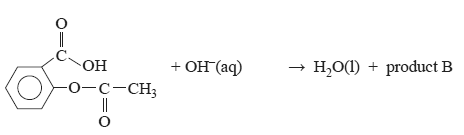Draw the structure of product B Solution9) The boiling points of several alkanols are provided in the following table.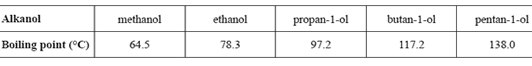A mixture of two of these alkanols is to be separated in an experiment using fractional distillation. The mixture is placed into the distillation apparatus at room temperature and then gently heated. The first fraction is collected at 97.2 °C. a. i. Identify one alkanol that could not be present in this mixture. Solution ii. By specifically referring to this experiment, explain why the alkanol identified in part i. could not be present. (1 + 1 = 2 marks) Solution b. Provide one reason why the distillation flask should not be heated using a bunsen burner. (1 mark) Solution c. Butane and propan-1-ol have similar molar masses. The boiling point of butane is –138.4 °C and that of propan-1-ol is 97.2 °C. Explain, in terms of intermolecular forces, the difference between the boiling points of these two compounds. (3 marks) Solution Click for the assessor's comments